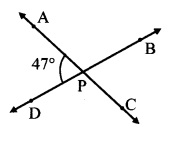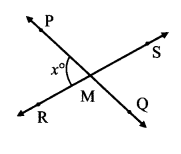# Maharashtra Board Class 7 Maths Solutions Chapter 4 Angles and Pairs of Angles Practice Set 20

## Maharashtra State Board Class 7 Maths Solutions Chapter 4 Angles and Pairs of Angles Practice Set 20

Question 1.
Lines AC and BD intersect at point P. m∠APD = 47° Find the measures of ∠APB, ∠BPC, ∠CPD.Solution:
∠APD and ∠APB are angles in a linear pair.
∴m∠APD + m∠APB = 180°
∴47 + m∠APB = 180
∴47 + m∠APB – 47 = 180 – 47 ….(Subtracting 47 from both sides)
∴m∠APB = 133°
m∠CPD = m∠APB = 133° … .(Vertically opposite angles)
m∠BPC = m∠APD = 47° … .(Vertically opposite angles)
∴The measures of ∠APB, ∠BPC and ∠CPD are 133°, 47° and 133° respectively.

Question 2.
Lines PQ and RS intersect at point M. m∠PMR = x°.What are the measures of ∠PMS, ∠SMQ and ∠QMR?Solution:
∠PMR and ∠PMS are angles in a linear pair.
∴ m∠PMR + m∠PMS = 180°
∴ x + m∠PMS = 180
∴ m∠PMS = (180-x)°
m∠QMR = m∠PMS = (180 – x)° … .(Vertically opposite angles)
m∠SMQ = m∠PMR = x° …. (Vertically opposite angles)
∴The measures of ∠PMS, ∠SMQ and ∠QMR are (180 – x)°, x° and (180 – x)° respectively.